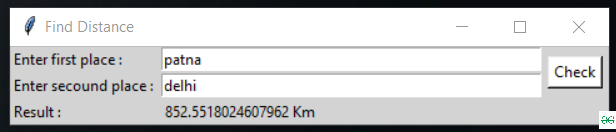# Build a GUI Application to get distance between two places using Python

Prerequisite: Tkinter

In this article, we are going to write a python script to get the distance between two places and bind it with the GUI application. To install GeoPy module, run the following command in your terminal.

pip install geopy

Approach used:

• Import the geopy module.
• Initialize Nominatim API to get location from the input string.
• Get latitude and longitude with geocode().
• Get the distance with distance() method in geopy.

Implementation:

## Python3

 `# import module ` `from` `geopy.geocoders ``import` `Nominatim ` `from` `geopy ``import` `distance ` ` `  `# initialize Nominatim API ` `geolocator ``=` `Nominatim(user_agent``=``"geoapiExercises"``) ` ` `  `# place input ` `Input_place1 ``=` `"delhi"` `Input_place2 ``=` `"patna"` ` `  `# Get location of the input strings ` `place1 ``=` `geolocator.geocode(Input_place1) ` `place2 ``=` `geolocator.geocode(Input_place2) ` ` `  `# Get latitude and longitude ` `Loc1_lat, Loc1_lon ``=` `(place1.latitude), (place1.longitude) ` `Loc2_lat, Loc2_lon ``=` `(place2.latitude), (place2.longitude) ` ` `  `location1 ``=` `(Loc1_lat, Loc1_lon) ` `location2 ``=` `(Loc2_lat, Loc2_lon) ` ` `  `# display the distance ` `print``(distance.distance(location1, location2).km, ``" kms"``) `

Output:

```852.5518024607962  kms
```

GUI Application to Measure Distance with Tkinter:

## Python3

 `# import modules ` `from` `tkinter ``import` `*` `from` `geopy.geocoders ``import` `Nominatim ` `from` `geopy ``import` `distance ` ` `  `# user defined funtion ` `def` `get_dis(): ` `    ``try``: ` `         `  `        ``geolocator ``=` `Nominatim(user_agent``=``"geoapiExercises"``) ` `         `  `        ``place1 ``=` `geolocator.geocode(``str``(e1.get())) ` `        ``place2 ``=` `geolocator.geocode(``str``(e2.get())) ` ` `  ` `  `        ``Loc1_lat,Loc1_lon ``=` `(place1.latitude),(place1.longitude) ` `        ``Loc2_lat,Loc2_lon ``=` `(place2.latitude),(place2.longitude) ` ` `  `        ``location1``=``(Loc1_lat,Loc1_lon) ` `        ``location2``=``(Loc2_lat,Loc2_lon) ` ` `  `        ``res ``=` `(``str``(distance.distance(location1, location2).km)``+``" Km"``) ` ` `  `        ``result.``set``(res) ` `    ``except``: ` `        ``result.``set``(``"someting went wrong"``) ` ` `  `# object of tkinter ` `# with background set to light grey ` `master ``=` `Tk() ` `master.configure(bg``=``'light grey'``) ` `master.title(``"Find Distance"``) ` ` `  `# Variable Classes in tkinter ` `result ``=` `StringVar(); ` ` `  ` `  `# Creating label for each information ` `# name using widget Label  ` `Label(master, text``=``"Enter first place : "` `, bg ``=` `"light grey"``).grid(row``=``1``, sticky``=``W) ` `Label(master, text``=``"Enter secound place : "` `, bg ``=` `"light grey"``).grid(row``=``2``, sticky``=``W) ` ` `  `Label(master, text``=``"Result :"``, bg ``=` `"light grey"``).grid(row``=``3``, sticky``=``W) ` ` `  `# Creating label for class variable ` `# name using widget Entry ` `Label(master, text``=``"``", textvariable=result,bg = "``light grey").grid(row``=``3``,column``=``1``, sticky``=``W) ` ` `  ` `  `e1 ``=` `Entry(master,width ``=` `50``) ` `e1.grid(row``=``1``, column``=``1``) ` `e2 ``=` `Entry(master,width ``=` `50``) ` `e2.grid(row``=``2``, column``=``1``) ` ` `  `# creating a button using the widget   ` `b ``=` `Button(master, text``=``"Check"``, command``=``get_dis, bg ``=` `"white"``) ` `b.grid(row``=``1``, column``=``2``,columnspan``=``2``, rowspan``=``2``,padx``=``5``, pady``=``5``,) ` ` `  `mainloop() `

Output:Attention geek! Strengthen your foundations with the Python Programming Foundation Course and learn the basics.

To begin with, your interview preparations Enhance your Data Structures concepts with the Python DS Course.

My Personal Notes arrow_drop_upCheck out this Author's contributed articles.

If you like GeeksforGeeks and would like to contribute, you can also write an article using contribute.geeksforgeeks.org or mail your article to contribute@geeksforgeeks.org. See your article appearing on the GeeksforGeeks main page and help other Geeks.

Please Improve this article if you find anything incorrect by clicking on the "Improve Article" button below.

Article Tags :

Be the First to upvote.

Please write to us at contribute@geeksforgeeks.org to report any issue with the above content.